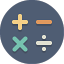Calculator

Features

A multipurpose calculator that has the following features:
1. All basic operations such as:
2. subtraction
3. multiplication
4. division
5. modulus (mod)
6. power of (x^y)
7. percentage
over integers, decimal numbers and complex numbers.
2. Memory variables that can be used as values to evaluate expressions. You can directly add/subtract/store to a memory variable.
3. Trigonometric functions such as sin, cos, tan, sinh, cosh, tanh and their inverses over integers, decimals and complex numbers.
4. Permutations, combinations and factorial for integers, decimals and complex numbers.
5. Logarithm functions log (log to base 10) and ln (log to base e) over integers, decimals and complex numbers.
6. Ability to use the answer of previous evaluation in an expression multiple times. For ex. 2*Ans+3/Ans